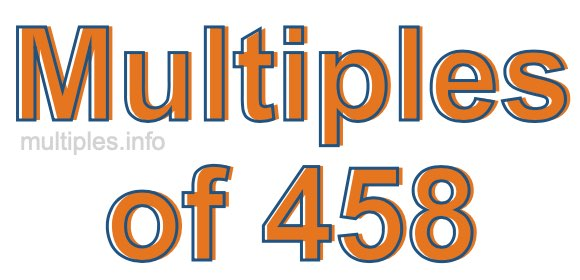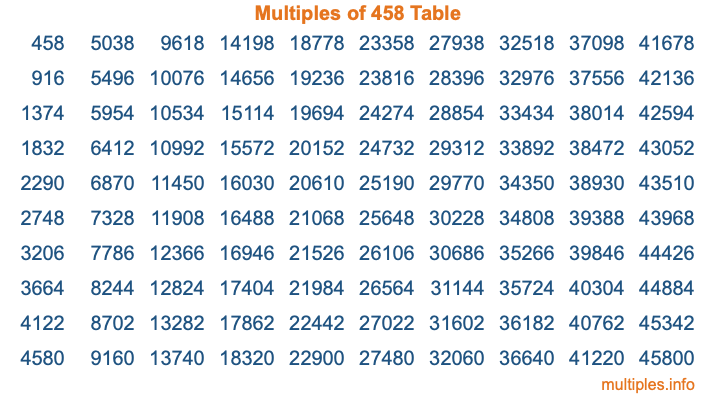Multiples of 458Welcome to the Multiples of 458 page. Here we will first teach you everything you will ever need to know about the multiples of 458, and then give you a study guide summary of everything we taught you to make sure you remember it all. Use this page to look up facts and learn information about the multiples of 458. This page will make you a multiples of four hundred fifty-eight expert!

Definition of Multiples of 458
Multiples of 458 are all the numbers that when divided by 458 equal an integer. Each of the multiples of 458 are called a multiple. A multiple of 458 is created by multiplying 458 by an integer.

Therefore, to create a list of multiples of 458, you start with 1 multiplied by 458, then 2 multiplied by 458, then 3 multiplied by 458, and so on for as long as you want. Thus, the list of the first five multiples of 458 is 458, 916, 1374, 1832, and 2290. To see a larger list of multiples of 458, see the printable image of Multiples of 458 further down on this page. We also have a category where you can choose any nth multiple of 458.

Multiples of 458 Checker
The Multiples of 458 Checker below checks to see if any number of your choice is a multiple of 458. In other words, it checks to see if there is any number (integer) that when multiplied by 458 will equal your number. To do that, we divide your number by 458. If the the quotient is an integer, then your number is a multiple of 458.

Is  a multiple of 458?

Least Common Multiple of 458 and ...
A Least Common Multiple (LCM) is the lowest multiple that two or more numbers have in common. This is also called the smallest common multiple or lowest common multiple and is useful to know when you are adding our subtracting fractions. Enter one or more numbers below (458 is already entered) to find the LCM.

Check out our LCM Calculator if you need more details about the Least Common Multiple or if you need the LCM for different numbers for adding and subtraction fractions.

nth Multiple of 458
As we stated above, 458 is the first multiple of 458, 916 is the second multiple of 458, 1374 is the third multiple of 458, and so on. Enter a number below to find the nth multiple of 458.

th multiple of 458

Multiples of 458 vs Factors of 458
458 is a multiple of 458 and a factor of 458, but that is where the similarities end. All postive multiples of 458 are 458 or greater than 458. All positive factors of 458 are 458 or less than 458.

Below is the beginning list of multiples of 458 and the factors of 458 so you can compare:

Multiples of 458: 458, 916, 1374, 1832, 2290, etc.

Factors of 458: 1, 2, 229, 458

As you can see, the multiples of 458 are all the numbers that you can divide by 458 to get a whole number. The factors of 458, on the other hand, are all the whole numbers that you can multiply by another whole number to get 458.

It's also interesting to note that if a number (x) is a factor of 458, then 458 will also be a multiple of that number (x).

Multiples of 458 vs Divisors of 458
The divisors of 458 are all the integers that 458 can be divided by evenly. Below is a list of the divisors of 458.

Divisors of 458: 1, 2, 229, 458

The interesting thing to note here is that if you take any multiple of 458 and divide it by a divisor of 458, you will see that the quotient is an integer.

Multiples of 458 Table
Below is an image of the first 100 multiples of 458 in a table. The table is in chronological order, column by column. The first column has the first ten multiples of 458, the second column has the next ten multiples of 458, and so on.The Multiples of 458 Table is also referred to as the 458 Times Table or Times Table of 458. You are welcome to print out our table for your studies.

Negative Multiples of 458
Although not often discussed or needed in math, it is worth mentioning that you can make a list of negative multiples of 458 by multiplying 458 by -1, then by -2, then by -3, and so on, to get the following list of negative multiples of 458:

-458, -916, -1374, -1832, -2290, etc.

Multiples of 458 Summary
Below is a summary of important Multiples of 458 facts that we have discussed on this page. To retain the knowledge on this page, we recommend that you read through the summary and explain to yourself or a study partner why they hold true.

There are an infinite number of multiples of 458.

A multiple of 458 divided by 458 will equal a whole number.

458 divided by a factor of 458 equals a divisor of 458.

The nth multiple of 458 is n times 458.

The largest factor of 458 is equal to the first positive multiple of 458.

458 is a multiple of every factor of 458.

458 is a multiple of 458.

A multiple of 458 divided by a divisor of 458 equals an integer.

458 divided by a divisor of 458 equals a factor of 458.

Any integer times 458 will equal a multiple of 458.

Multiples of a Number
Here you can get the multiples of another number, all with the same attention to detail as we did for multiples of 458 on this page.

Multiples of
Multiples of 459
Did you find our page about multiples of four hundred fifty-eight educational? Do you want more knowledge? Check out the multiples of the next number on our list!